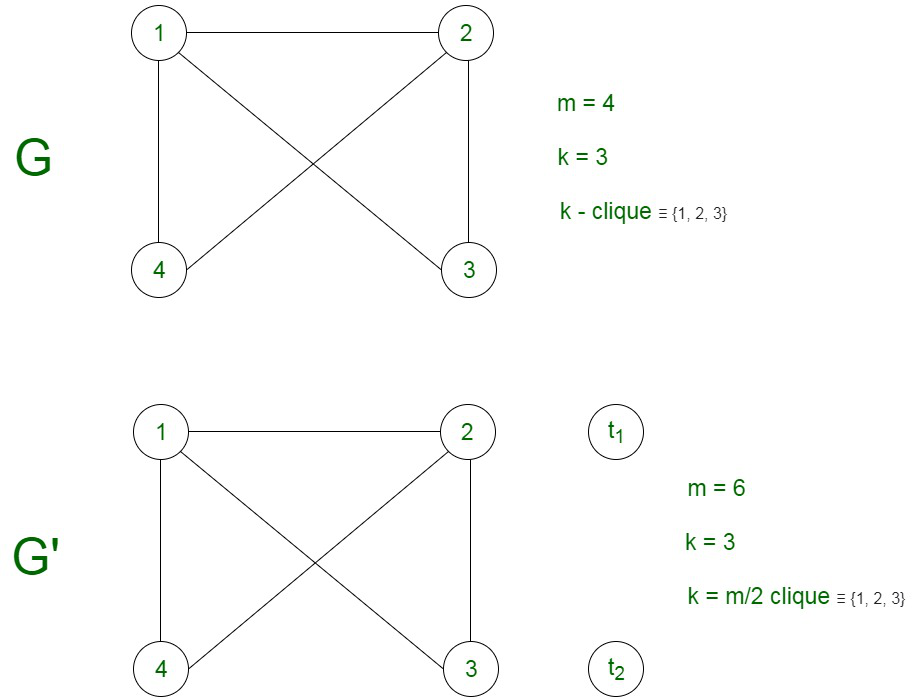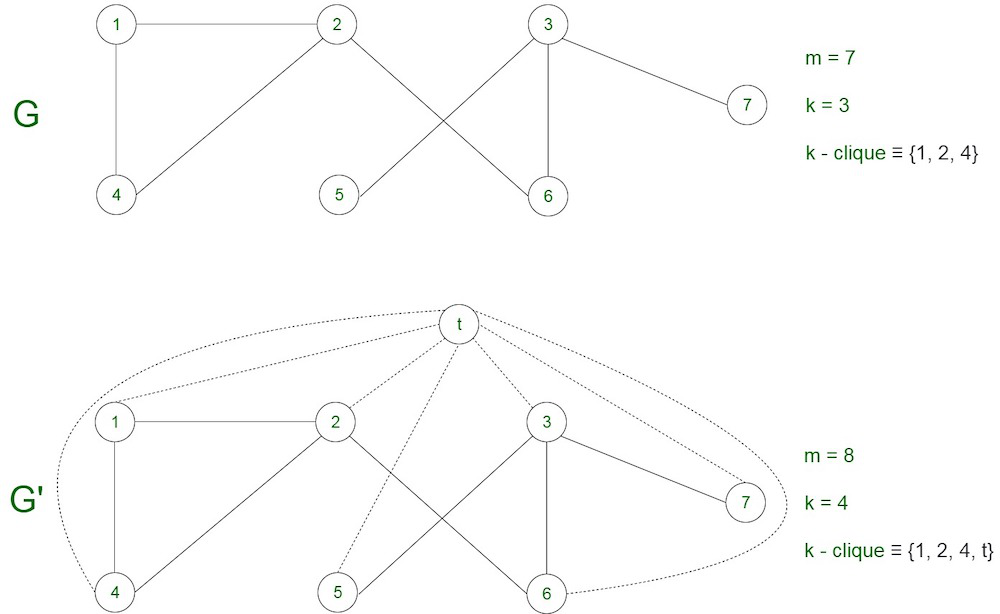Open in App
Not now

# Half clique NP Complete problem

• Last Updated : 13 Oct, 2022

A half clique in a graph is a set of n/2 vertices such that each vertex shares an edge with every other vertex, that is, the k = n/2 vertices of the graph form a complete graph.
Problem – Given a graph G(V,E) ,the problem is to determine if the graph contains a clique of size atleast k = |V/2|.
Explanation-
An instance of the problem is an input specified to the problem. An instance of the Half-Clique problem is a graph G (V, E) and a positive integer k, and the problem is to check whether a clique of size k = |V/2| exists in G. Since an NP Complete problem, by definition, is a problem which is both in NP and NP hard, the proof for the statement that a problem is NP Complete consists of two parts:

1. Half – Clique Problem is in NP :
If any problem is in NP, then, given a ‘certificate’, which is a solution to the problem and an instance of the problem (a graph G and a positive integer k, in this case), we will be able to verify (check whether the solution given is correct or not) the certificate in polynomial time.
The certificate is a subset V’ of the vertices, which comprises the vertices belonging to the half-clique. We can validate this solution by checking that each pair of vertices belonging to the solution are adjacent, by simply verifying that they share an edge with each other. This can be done in polynomial time, that is O(V +E) using the following strategy of graph G(V,E):

```flag=true
Count the number of vertices in the subset V'
If not equal to V/2 :
flag = false
Else :
For every pair {u,v} in the subset V’:
Check that these two vertices {u,v} share an edge
If there is no edge ,set flag to false and break
If flag is true:
Solution is correct
Else:
Solution is incorrect ```

2. Half-Clique Problem is NP Hard :
To prove that the half-clique problem is NP Hard, we take the help of a problem which is already NP Hard and show that this problem can be reduced to the half-clique problem. For this, we consider the Clique problem, which is NP Complete (and hence NP Hard).
Every instance of the clique problem consisting of the graph G (V,E) and an integer k can be converted to the required graph G’ (V’,E’) and k’ of the half clique problem. The deduction that can be made is that the graph G’ will have a clique of size n/2 , if the graph G has a clique of size k. Let m be the number of nodes in the graph G. We will now prove that the problem of computing the clique indeed boils down to the computation of the independent set. The reduction can be proved by the following two propositions:

• If k > = m/2, then for a constant number t, we add t nodes each of degree 0, for a graph G’. The graph G’ has a total number of nodes equivalent to n = m + t, that is, the summation of all the nodes of graph G along with the extra nodes, such that it is equivalent to 2k, for any arbitrary value of k. Now k = n/2. This can be done by taking t = 2k -m. Then, the graph G has a clique of size k if and only if the graph G’ has a clique of size k.• If k < m/2 , then we add t additional nodes for the creation of graph G’. Edges can also be added from each new node to every other node in the graph.  Therefore, any k-clique in G, for any arbitrary value of k combines with the t new nodes to make a (k+t)-clique in G’, since edges have been added between each pair of vertices. A k+t-sized clique in G’ must include at least k old nodes, which form a clique in the graph G. Therefore, the value of t is picked such that k+t = (m+t)/2, or t = m-2k, which makes the clique size in G’ equivalent to n/2 exactly.My Personal Notes arrow_drop_up
Related Articles